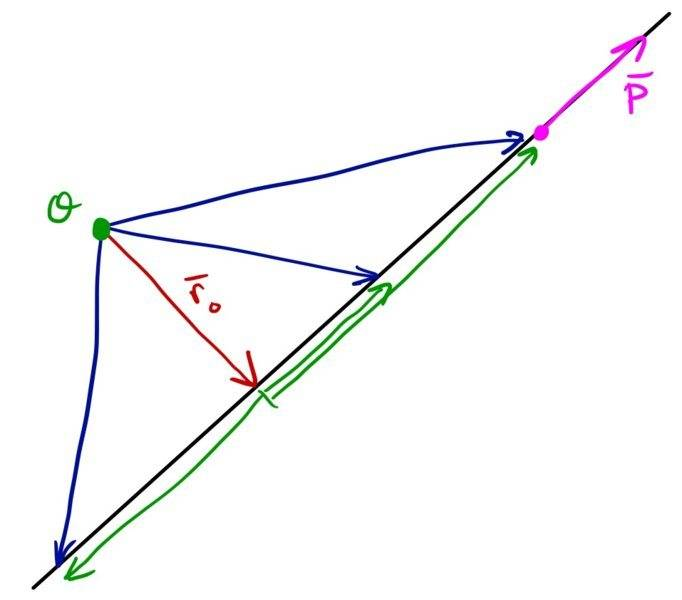# Angular momentum of particle about the origin

Gold Member

## Homework Statement

A particle (5 kg) moves with constant velocity 2 m/s along the straight line 2y=3x+4, the angular momentum of the particle about origin is?

L=r x p

## The Attempt at a Solution

For a 2d problem we take the component of velocity perpendicular to the point about which we want to find the momentum and multiply it with the perpendicular distance. Here slope of the line gives tanθ=3/2, p=mv=10 and L=mvrcosθ, mvcosθ equals 20/√13 but isn't the distance r (here r=the y coordinate of the particle) continuously increasing? I get the correct answer for r=2 though, I don't understand how. I'd really appreciate some help, thank you

Orodruin
Staff Emeritus
Homework Helper
Gold Member
2021 Award
##\vec r \times \vec p## only depends on the part of ##\vec r## that is orthogonal to ##\vec p##.

Gold Member
##\vec r \times \vec p## only depends on the part of ##\vec r## that is orthogonal to ##\vec p##.
I know only the very basics of vectors, (its yet to be reached in our mathematics coursework). I suppose the part of r that's perpendicular to momentum is what you mean but that part seems to be increasing with time as well

Orodruin
Staff Emeritus
Homework Helper
Gold Member
2021 Award
but that part seems to be increasing with time as well
Why do you think so? (It is not correct)

Orodruin
Staff Emeritus
Homework Helper
Gold Member
2021 AwardHere is a quick drawing of the situation.
The red vector is always the part of the position vector orthogonal to p. The blue vectors are different position vectors for the part let moving along the black line and the magenta vector its momentum. The green vectors are the components of the position vectors not perpendicular to p.

#### Attachments

Gold Member
View attachment 232643
Here is a quick drawing of the situation.
The red vector is always the part of the position vector orthogonal to p. The blue vectors are different position vectors for the part let moving along the black line and the magenta vector its momentum. The green vectors are the components of the position vectors not perpendicular to p.
Ohh, I understand really well where I was thinking wrong. The diagram helped a lot. Thank you :D
The fact that people on this website take out so much time to help students like me amazes me, so I've thought about donating to the site from my savings after my exams this year are over. can you direct me to where I can do that?

Orodruin
Staff Emeritus
•